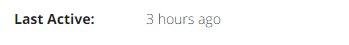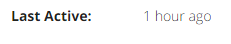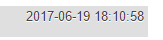# Show nice time formats (now, 15 minutes, 2 hours ago etc)

I have the following function that converts a timestamp from `YYYY-MM-DD HH:MM:SS` to a nicer format like an hour ago or last week etc.

Example:``````////////////////////////////////////////////////////////////////////////////////////////////////////////
// FUNCTION TO TRANSLATE DATE AND TIMES INTO NICE DATE EG 2 HOURS AGO ETC...
function nicetime(\$date)
{
if(empty(\$date)) {
return "No date provided";
}

\$periods         = array("second", "minute", "hour", "day", "week", "month", "year", "decade");
\$lengths         = array("60","60","24","7","4.35","12","10");

\$now             = time();
\$unix_date       = strtotime(\$date);

// check validity of date
if(empty(\$unix_date)) {
}

// is it future date or past date
if(\$now > \$unix_date) {
\$difference     = \$now - \$unix_date;
\$tense         = "ago";

} else {
\$difference     = \$unix_date - \$now;
\$tense         = "from now";
}

for(\$j = 0; \$difference >= \$lengths[\$j] && \$j < count(\$lengths)-1; \$j++) {
\$difference /= \$lengths[\$j];
}

\$difference = round(\$difference);

if(\$difference != 1) {
\$periods[\$j].= "s";
}

return "\$difference \$periods[\$j] {\$tense}";
}
``````

At the moment, the smallest time it will give is one hor ago. How can I change it so that it displays now if in the last few minutes or 15 minutes if within 15 minutes etc?

Did you write this function yourself?

No, I added the fallbacks so if there isn’t a date set it will return ‘no date provided’ and if the date has been set by default (ie a bunch of zeros) it displays bad date - but then they are hidden in with PHP on the page template if they return either of those values.

I think the bulk of the function came from posts on the W3 and StackOverflow if I rember correctly.

Here is a quick fix to get what you’re looking for. Just determine if difference is less than 15 minutes, which using unix would be

``````60 * 15
``````

So use this right after your last calculation of difference and terms

``````// is it future date or past date OR NOW
if(\$now > \$unix_date) {
\$difference     = \$now - \$unix_date;
\$tense         = "ago";

} else {
\$difference     = \$unix_date - \$now;
\$tense         = "from now";
}

if( \$difference < (60*15) ) {
\$difference = null;
\$tense = "now";
}
``````

Thanks - just tried the tweak but it still seems to be showing 1 hour ago.Timestamp in DB reads 18:10 and I’ve tested at 18:14.

Is it a time zone issue, or a daylight savings time thing? For example, is the database time GMT and the current time BST which is an hour later, so in fact it’s correct because the difference is 1h4m.

No, I checked that out. The timestamp reads 18:10 - thats when i logged in to the account - I checked whether the conversion to ‘nice time’ had happened at 18:14 and then again at 18:30.

Checking it again now - at 18:48 - the nice time now displays 2 hours ago - it should say 48 minutes ago. Definately shouldn’t be saying 2 hours.

It seems to be starting at 1 hour when the login is made rather than a minute.

I can show you a screenshot of it working in a PHP test, though I think the function is poorly constructed and difficult to work in lacking documentation. This was just a quick fix.

The function lacks any documentation on the type of date the parameter should be. I had to look it up all up myself.

It’s a good start, and certainly useful. I wish to refactor it a bit. I will share what I come up with.

Am I wrong or is your first length index of “60” unnecessary because the timestamp result is already in seconds. Does the for loop not unnecessarily divide by seconds when it is already seconds? Just trying to understand how that for loop logic works.

Yes, the timestamp in the DB is in seconds.I’m not sure what the loop logic is doing but it is starting at an hour and seems to counting from there. So, effectively I think it is skipping the first hour.

Gotcha.

So here is what I am getting. The function converts a timestamp into a unix time, and uses that only for all calculations. With that in mind I am working on establishing some consistency here.

Here is what I am getting using the following values:

``````Now: 1497898883                             // time()
Date: 2017-06-19 01:00:00                  // Static Timestamp
Time: 1497848400                          // strtotime() of Date
Nice(): 14 minutes ago                   // Result of nicetime()
Difference: 50483                       // Now > Time ? Now - Time : Time - Now
// Skip Diff Seconds (Unix is in seconds above)
Diff Minutes: 841.38333333333         // Difference / 60 (Because 60 seconds in minute)
Hours: 14.023055555556               // (Difference / 60) / 60 (Because 60 minutes in hour)
Days: 1.168587962963                // ((Difference 60) / 60) / 12) (Because 12 hours in a day)
``````

Right - thanks.

But I don’t appear to be getting anything less than the hour for nicetime(). How are you seeing 14 minutes?

Now following the pattern above, here is what the loop does

``````\$lengths = array("60","60","24","7","4.35","12","10");
\$difference = 50483;

// for each index of \$lengths as \$index while \$difference > \$index

\$difference / 60;
\$difference = 841.383333333;

\$difference / 60;
\$difference = 14.023055556;

// Because 14 is less than 24 (next index to divide by) stop here

// Returns "14 minutes ago."

``````

I’m not. This was all done by hand using the logic in the function. See my next comment using the for loop logic applied to my manual calculations above.

I carried the difference I determined between the two UNIX times above and brought it into the function’s loop.

So my point in all this is to say, that determining the period by if the UNIX difference (in seconds) is greater than the next divisible length is not a way to determine its’ period.

What you want to get to is a point where my initial fix makes sense. If you are calculating only in seconds, you can simply determine whether \$difference is less than 15 minutes which would be the result of (60*15).

I would suggest making a separate function that determines time periods, and use that in this function. For example if you are always going to be calculating in UNIX seconds.

Ah ok. Thanks a lot for your help here.

I think I understand the logic behind what you’ve done - well the maths of it at least - not sure how I would rework to functiuon to work as I had originally intended.

I’m getting some results:

``````Difference: 22986 seconds
Figuring 22986 / 60 = 383 minutes
Figuring 383.1 / 60 = 6 hours
6 hours ago
``````

Give this a shot?

``````function nicetime(\$date) {

// Validate
if( !isset(\$date) && !strtotime(\$date) ) {
return "Improper Parameter.";
} else {}

// Variables
\$now = time();
\$date = strtotime(\$date);

\$periods = array(
array("second", 1),
array("minute", 60),
array("hour", 60),
array("day", 24),
array("week", 7),
array("month", 4.35),
array("year", 12)
);

// Future or Past
if( \$now > \$date ) {
\$difference = \$now - \$date;
\$tense = "ago";
} else {
\$difference = \$date - \$now;
\$tense = "from now";
}

// Present
if( \$difference < 900 ) { \$tense = "now"; }

// Debug / Testing
echo "Difference: ".\$difference." ".\$periods."s<br/>";

// Safe Variable to Calculate
\$figure = \$difference;

// Calculate
for( \$index = 1; (\$figure >= 1 && (\$figure / \$periods[\$index]) >= 1) && \$index < count(\$periods); \$index++ ) {
// Debug / Testing
echo "Figuring ".\$figure." / ".\$periods[\$index];

// Figure
\$figure /= \$periods[\$index];

// Plurality Check
if( \$figure != 1) { \$periods[\$index].="s"; }

// Debug / Testing
echo " = ".round(\$figure)." ".\$periods[\$index]."<br/>";
}

// Result
echo round(\$figure)." ".\$periods[\$index-1]." ".\$tense;
}
``````

Quick fix. It should report only “now” when within 15 minutes. Instead of something like “12 minutes now”.

``````function nicetime(\$date) {

// Validate
if( !isset(\$date) && !strtotime(\$date) ) {
return "Improper Parameter.";
} else {}

// Variables
\$now = time();
\$date = strtotime(\$date);

\$periods = array(
array("second", 1),
array("minute", 60),
array("hour", 60),
array("day", 24),
array("week", 7),
array("month", 4.35),
array("year", 12)
);

// Future or Past
if( \$now > \$date ) {
\$difference = \$now - \$date;
\$tense = "ago";
} else {
\$difference = \$date - \$now;
\$tense = "from now";
}

// Present
if( \$difference < 900 ) { return "now"; }

// Debug / Testing
echo "Difference: ".\$difference." ".\$periods."s<br/>";

// Safe Variable to Calculate
\$figure = \$difference;

// Calculate
for( \$index = 1; (\$figure >= 1 && (\$figure / \$periods[\$index]) >= 1) && \$index < count(\$periods); \$index++ ) {
// Debug / Testing
echo "Figuring ".\$figure." / ".\$periods[\$index];

// Figure
\$figure /= \$periods[\$index];

// Plurality Check
if( \$figure != 1) { \$periods[\$index].="s"; }

// Debug / Testing
echo " = ".round(\$figure)." ".\$periods[\$index]."<br/>";
}

// Result
return round(\$figure)." ".\$periods[\$index-1]." ".\$tense;
}
``````
1 Like

Now as to your original question, how can you make this return smaller values?

From this section in the new function

``````// Present
if( \$difference < 900 ) { return "now"; }

elseif( \$difference < 1800 ) { return "recently"; }
``````

You can also use the PHP DateTime interface.

Example:

``````\$date = new DateTime("10pm");
\$now = new DateTime("now");
echo "<br/><br/>It is ".\$now->diff(\$date)->format("%h hours and %i minutes")." till then<br/><br/>.";
// It is 1 hours and 15 minutes till then
``````

Might be able to combine the function with this determing if a time needs plural or not.

1 Like

Like this?

``````// Present
if( \$difference < 900 ) { return "now"; }